### All 9 results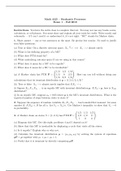#### Stochastic Processes (Exams 1, 2.a, 2.b)

(0)
\$16.48

Questions and answers for exams on the stochastic process. The course is designed to introduce undergraduates to the fundamental ideas of stochastic (or random) processes. Topics to be covered are Markov chains (discrete and continuous time), renewal theory, queueing theory, Brownian motion, and stationary processes.

• Bundle
•  • 3 items •
• by RajR •
i x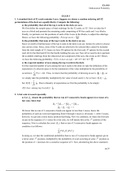#### Probability Exams (1- 3)

(0)
\$20.48

Probability exams testing basic theorems of probability, permutations and combinations, binomial and multinomial theorems, random variables with densities, sequences of independent identically distributed random variables, method of moments, the moment-generating function, central limit theorem, and standard-type probability distributions.

• Bundle
•  • 3 items •
• by RajR •
i x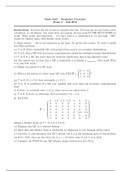#### Exam 2.a

(0)
\$7.48

This course is designed to introduce the undergraduate to the fundamental ideas of stochastic (or random) processes. Such processes are used in the study of mathematical models where there are elements of uncertainty and hence probabilistic quantities are introduced into the model. These models are found in fields such as the analysis of algorithms, the theory of queues, the pricing of stock options, financial mathematics, econometrics, linear programming, and biomathematics. The course will cov...

•  Bundle
• Exam
•  • 5 pages •
• by RajR •
Quick View
i x
•
•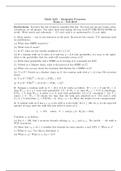#### Exam 2.b

(0)
\$7.48

This course is designed to introduce the undergraduate to the fundamental ideas of stochastic (or random) processes. Such processes are used in the study of mathematical models where there are elements of uncertainty and hence probabilistic quantities are introduced into the model. These models are found in fields such as the analysis of algorithms, the theory of queues, the pricing of stock options, financial mathematics, econometrics, linear programming, and biomathematics. The course will cov...

•  Bundle
• Exam
•  • 3 pages •
• by RajR •
Quick View
i x
•
•#### Exam 1

(0)
\$8.48

This course is designed to introduce the undergraduate to the fundamental ideas of stochastic (or random) processes. Such processes are used in the study of mathematical models where there are elements of uncertainty and hence probabilistic quantities are introduced into the model. These models are found in fields such as the analysis of algorithms, the theory of queues, the pricing of stock options, financial mathematics, econometrics, linear programming, and biomathematics. The course will cov...

•  Bundle
• Exam
•  • 5 pages •
• by RajR •
Quick View
i x
•
•#### Final Exam

(0)
\$10.48

This course is an introduction to the theory of probability. Topics to be included are basic theorems of probability, permutations and combinations, binomial and multinomial theorems, random variables with densities, sequences of independent identically distributed random variables, method of moments, the moment-generating function, central limit theorem, and standard-type probability distributions.

•  Bundle
• Exam
•  • 12 pages •
• by RajR •
Quick View
i x
•
•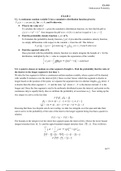#### Exam 2

(0)
\$7.48

This course is an introduction to the theory of probability. Topics to be included are basic theorems of probability, permutations and combinations, binomial and multinomial theorems, random variables with densities, sequences of independent identically distributed random variables, method of moments, the moment-generating function, central limit theorem, and standard-type probability distributions.

•  Bundle
• Exam
•  • 10 pages •
• by RajR •
Quick View
i x
•
•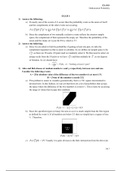#### Exam 1

(0)
\$8.48

This course is an introduction to the theory of probability. Topics to be included are basic theorems of probability, permutations and combinations, binomial and multinomial theorems, random variables with densities, sequences of independent identically distributed random variables, method of moments, the moment-generating function, central limit theorem, and standard-type probability distributions